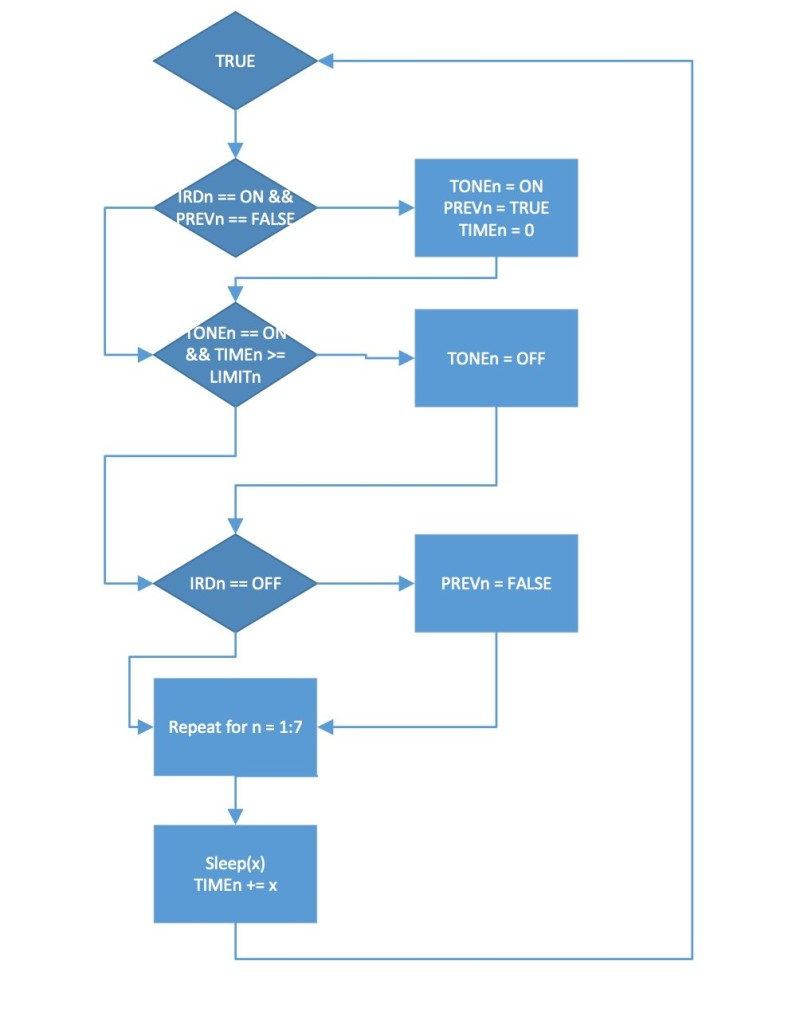# Project work3:Arduino code and the circuit diagram using arduino

In order to realise the “music garden”,the most important part is the Arduino code and circuit diagram.

First of all,I will present my Arduino code:(I will show you the way I think as a flow chart at first)

Flow Chart:Details:this flow chart is a mode for one single flower pot

IRDs means the sensor

PREVn means the records about whether there are people in front the sensor

When turn on the IRDn and the PREVn equals FALSE,Which will trigger the sound card to play tone(TONEn)，then set the PREVn equals TRUE,and time(TIMEn) set up to 0,and that cycle repeats.

Code:

const int sets = 8;
const int tick = 100;
int IRD[sets] = {30, 31, 32, 33, 34, 35, 36, 37};
int music[sets] = {38, 39, 40, 41, 42, 43, 44, 45};
bool previous[sets] = {false, false, false, false, false, false, false, false};
double limit[sets] = {2.0, 2.0, 2.0, 2.0, 2.0, 2.0, 2.0, 2.0};
double time[sets] = {0.0, 0.0, 0.0, 0.0, 0.0, 0.0, 0.0, 0.0};

void setup() {
// put your setup code here, to run once:
for (int i = 0; i < sets; ++i) {
pinMode(IRD[i], INPUT);
pinMode(music[i], OUTPUT);
digitalWrite(music[i], HIGH);
}
}

void loop() {
// put your main code here, to run repeatedly:
int val;
for (int i = 0; i < sets; ++i) {
if (val == LOW && !previous[i]) {
digitalWrite(music[i], LOW);
previous[i] = true;
time[i] = 0.0;
}

if (val == LOW && time[i] >= limit[i]) {
digitalWrite(music[i], HIGH);
}
if (val == HIGH) {
previous[i] = false;
digitalWrite(music[i], HIGH);}}delay(tick);
for (int i = 0; i < sets; ++i) {
time[i] += (double)tick/1000;
}
}

Secondly:I will present my circuit diagram

In practice,I did not obey strictly according to this diagram,I put all sound cards  and sensors together which will be easy for me to connect the circuit.

For more detail: Project work3:Arduino code and the circuit diagram